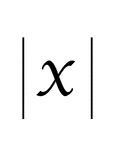### Flashcard of a math symbol for Absolute Value of X

A flashcard featuring a math symbol for Absolute Value of X### Flashcard of a math symbol for Approximately Equal To

A flashcard featuring a math symbol for Approximately Equal To### Flashcard of a math symbol for Congruent To

A flashcard featuring a math symbol for Congruent To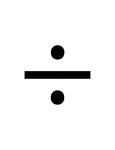### Flashcard of a math symbol for Division

A flashcard featuring a math symbol for Division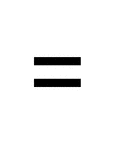### Flashcard of a math symbol for Equal To

A flashcard featuring a math symbol for Equal To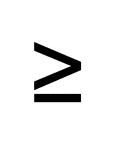### Flashcard of a math symbol for Greater Than or Equal To

A flashcard featuring a math symbol for Greater Than or Equal To### Flashcard of a math symbol for Less Than or Equal To

A flashcard featuring a math symbol for Less Than or Equal To### Flashcard of a math symbol for Multiplication

A flashcard featuring a math symbol for Multiplication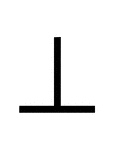### Flashcard of a math symbol for Perpendicular

A flashcard featuring a math symbol for Perpendicular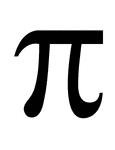### Flashcard of a math symbol for Pi

A flashcard featuring a math symbol for Pi### Flashcard of a math symbol for Subtraction

A flashcard featuring a math symbol for Subtraction### Flashcard of a math symbol for Sum

A flashcard featuring a math symbol for Sum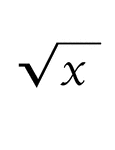### Flashcard of a math symbol for the Square Root of X

A flashcard featuring a math symbol for the Square Root of X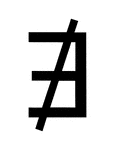### Flashcard of a math symbol for There Does Not Exist

A flashcard featuring a math symbol for There Does Not Exist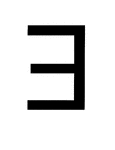### Flashcard of a math symbol for There Exists

A flashcard featuring a math symbol for There Exists### Flashcard of a math symbol for Therefore

A flashcard featuring a math symbol for Therefore